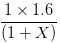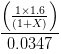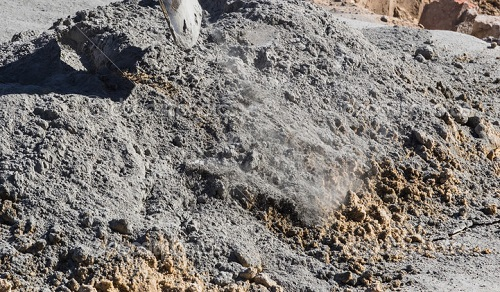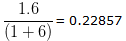Sorry, you do not have permission to ask a question, You must login to ask question. Become VIP Member

Do you need to remove the ads? Become VIP Member

## Quantity of Cement and Sand Calculation in Mortar

Quantity of cement mortar is required for rate analysis of brickwork and plaster or estimation of masonry work for a building or structure. Cement mortar is used in various proportions, i.e. 1:1, 1:2, 1:3, 1:4, 1:6, 1:8 etc.

### Calculation of quantity of cement mortar in brickwork and plaster:

For the calculation of cement mortar, let us assume that we use 1m3 of cement mortar. Procedure for calculation is:

1. Calculate the dry volume of materials required for 1m3 cement mortar. Considering voids in sands, we assume that materials consists of 60% voids. That is, for 1m3 of wet cement mortar, 1.6m3 of materials are required.

2. Now we calculate the volume of materials used in cement mortar based on its proportions.

Let’s say, the proportion of cement and sand in mortar is 1:X, where X is the volume of sand required.

Then, the volume of sand required for 1:X proportion of 1m3 cement mortar will be3. Volume of cement will be calculated as:Since the volume of 1 bag of cement is 0.0347 m3, so the number of bag of cement will be calculated as:Example:

For cement mortar of 1:6, the quantity calculated will be as below:

Sand quantity:Quantity of cement (in bags):

Volume of cement =There number of bags required == 6.58 bags.

## How to Properly Splice Column Reinforcement as per Indian Standards?

1. You are making us who are dealing with construction knowledgeable almost every day, keep it up

• Share
2.It was very simply explained and can comes to aid for field engineers. But the crux of this calculation is finding the voids, here i will explain you a simple and useful way to find the voids in sand.

Take a small container with uniform shape from bottom to top(say a dia of 15cm) and measure the depth of the container say X, now fill the container with sand using a trowel. After the container was filled completely, start pouring water and tap the sand with 12 mm rod uniformly all over the container till the water reaches the top of the container. Allow the sand in the container to settle for 10 minutes, now measure the distance above the sand and upto the top of the container say Y. Now we can get the percentage of voids with this simple formula (100/X)*Y.

Once we get the voids percentage we can calculate the cement and sand required for various cement mortars.

• Share
3.Thankx Bro……

• Share
4.thanks :)

• Share
5.its not for concrete , only for cement mortar.

• Share
6.wastage is how many percentage

• Share
7.very useful this formuls

• Share
8. cemen# sand iska equetion thoda parivarthan karsakthe. Eg:cement kithna sandal kithana

• Share
9.that was elementary math…
but apllication is different…..anywys tanxxx…was helpfull..

• Share
10.• Share
11.THANKS

• Share
12.excellent

• Share
•Where X is

• Share
13.thanks for this….how we can find out the quantity of sand and cement in 30m2 plastered area or 6m3 brick work?

• Share
14. This is very good. Please can u post the simple method on calculation of quantity of materials in concrete? thanks

• Share
15.16.